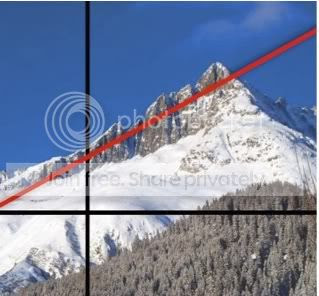## Sunday, January 2, 2011

### Slope is m? I don't get it!

Do we make learning math harder than it needs to be?

One of the "feature" concepts in first year algebra is the "slope-intercept" form of a linear equation, y=mx+b, where m is the slope and b represents the y-intercept. It seems to me we traditionally present this in some of the most counter-intuitive ways imaginable.First and most obviously, we represent two key values using letters that have no apparent association with those quantities.  Jeff Miller has undertaken a detailed study of the origin of the symbol m to denote slope. The consensus seems to be that it is not known why the letter m was chosen.*

Neither, so far as I can tell, is there any sort of agreement as to why the y-intercept is identified using the letter b. These seemingly arbitrary choices can be confusing to students looking for connections.

Further, if you consider that in a "real world" context slope defines the rate at which a quantity changes over time and that the y-intercept is an initial baseline value for that quantity, why don’t we teach the "intercept-slope" form of linear equations as y=b+mx (conceding the m and b), where we start with the initial value b which then changes according to the values of x and m. Would students not find this to be more intuitive than the traditionally taught form where change is being applied to a yet to be established quantity?Sometimes I think we're like medieval monks, reluctant to allow just anybody to share the "secret knowledge" we possess.Go to a previous post Multiplying on the Grid

*Weisstein, Eric W. "Slope." From MathWorld--A Wolfram Web Resource. http://mathworld.wolfram.com/Slope.html

#### 1 comment:

David said...

I teach my students y = mx + b because it is standard notation, at least in the US. They are familiar with the notation from previous math classes, and they will see it again in future math classes. The textbook uses the same notation. Web sites and study guides use the same notation.

Perhaps it's confusing to students that the symbols m and b are arbitrary, but it would be infinitely more confusing if every teacher chose his or her own notation.

NOTICE TO SPAM COMMENTERS
All comments to this blog are reviewed before being published. The chances of you getting a comment including ridiculously obvious "hidden" hyperlinks to porn sites or other spam published is virtually zero. So, save your time as well as mine, and take your tawdry business elsewhere.